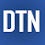## Pages

### Regression Example with XGBRegressor in Python

XGBoost stands for "Extreme Gradient Boosting" and it is an implementation of gradient boosting trees algorithm. The XGBoost is a popular supervised machine learning model with characteristics like computation speed, parallelization, and performance. You can find more about the model in this link.

In this post, we'll learn how to define the XGBRegressor model and predict regression data in Python. The tutorial covers:
1. Preparing the data
2. Defining and fitting the model
3. Predicting and checking the results
4. Video tutorial
5. Source code listing
We'll start by loading the required libraries. You may need to install them if they are not available on your machine.

```import xgboost as xgb
from sklearn.model_selection import train_test_split
from sklearn.model_selection import cross_val_score, KFold
from sklearn.metrics import mean_squared_error
import matplotlib.pyplot as plt ```

Preparing data

We use Boston house-price dataset as a regression dataset in this tutorial. After loading the dataset, first, we'll separate data into x - feature and y - label. Then we'll split them into the train and test parts. Here, I'll extract 15 percent of the dataset as test data.

```boston = load_boston()
x, y = boston.data, boston.target
xtrain, xtest, ytrain, ytest=train_test_split(x, y, test_size=0.15)```

Defining and fitting the model

For the regression problem, we'll use the XGBRegressor class of the xgboost package and we can define it with its default parameters. You can also set the new parameter values according to your data characteristics.

`xgbr = xgb.XGBRegressor(verbosity=0) `
`print(xgbr)`
```XGBRegressor(base_score=0.5, booster='gbtree', colsample_bylevel=1,
colsample_bynode=1, colsample_bytree=1, gamma=0,
importance_type='gain', learning_rate=0.1, max_delta_step=0,
max_depth=3, min_child_weight=1, missing=None, n_estimators=100,
reg_alpha=0, reg_lambda=1, scale_pos_weight=1, seed=None,
silent=None, subsample=1, verbosity=1)```

Next, we'll fit the model with train data.

`xgbr.fit(xtrain, ytrain)`

Predicting and checking the results

After training the model, we'll check the model training score.

`score = xgbr.score(xtrain, ytrain)  `
`print("Training score: ", score)`
`Training score:  0.9738225090795732 `

We can also apply the cross-validation method to evaluate the training score.

```scores = cross_val_score(xgbr, xtrain, ytrain,cv=10)
print("Mean cross-validation score: %.2f" % scores.mean())```
`Mean cross-validataion score: 0.87 `

Or if you want to use the KFlold method in cross-validation it goes as below.

```kfold = KFold(n_splits=10, shuffle=True)
kf_cv_scores = cross_val_score(xgbr, xtrain, ytrain, cv=kfold )
print("K-fold CV average score: %.2f" % kf_cv_scores.mean())```
`K-fold CV average score: 0.87`

Both methods show that the model is around 87 %  accurate on average.

Next, we can predict test data, then check the prediction accuracy. Here, we'll use MSE and RMSE as accuracy metrics.

```ypred = xgbr.predict(xtest)
mse = mean_squared_error(ytest, ypred)
print("MSE: %.2f" % mse)```
`MSE: 3.35`
`print("RMSE: %.2f" % (mse**(1/2.0)))`
`RMSE: 1.83 `

Finally, we'll visualize the original and predicted test data in a plot to compare visually.

```x_ax = range(len(ytest))
plt.plot(x_ax, ytest, label="original")
plt.plot(x_ax, ypred, label="predicted")```
`plt.title("Boston test and predicted data")`
```plt.legend()
plt.show()```

In this post, we've briefly learned how to build the XGBRegressor model and predict regression data in Python. The full source code is listed below.

Video tutorial

https://youtu.be/-D2Px4b0XQE

Source code listing

```import xgboost as xgb
from sklearn.model_selection import train_test_split
from sklearn.model_selection import cross_val_score, KFold
from sklearn.metrics import mean_squared_error
import matplotlib.pyplot as plt ```
` `
```boston = load_boston()
x, y = boston.data, boston.target
xtrain, xtest, ytrain, ytest = train_test_split(x, y, test_size=0.15)

xgbr = xgb.XGBRegressor(verbosity=0)
print(xgbr)

xgbr.fit(xtrain, ytrain)```
` `
`score = xgbr.score(xtrain, ytrain)   `
`print("Training score: ", score) `
` `
```# - cross validataion
scores = cross_val_score(xgbr, xtrain, ytrain, cv=5)
print("Mean cross-validation score: %.2f" % scores.mean())

kfold = KFold(n_splits=10, shuffle=True)
kf_cv_scores = cross_val_score(xgbr, xtrain, ytrain, cv=kfold )
print("K-fold CV average score: %.2f" % kf_cv_scores.mean())```
` `
```ypred = xgbr.predict(xtest)
mse = mean_squared_error(ytest, ypred)
print("MSE: %.2f" % mse)
print("RMSE: %.2f" % (mse**(1/2.0)))

x_ax = range(len(ytest))
plt.scatter(x_ax, ytest, s=5, color="blue", label="original")
plt.plot(x_ax, ypred, lw=0.8, color="red", label="predicted")
plt.legend()
plt.show()

```

1.Hello,
I've a couple of question.
1. What are labels for x and y axis in the above graph?

2. Then I’m trying to understand the following example.
I’m confused about the first piece of code. It seems to me that cross-validation and Cross-validation with a k-fold method are performing the same actions. In the second example just 10 times more. The result is the same. I dont understand the cross-validation in first example what is for?
Thanks,
Marco

2.Hi,
1. The plot describes 'medv' column of boston dataset (original and predicted). x label is the number of sample and y label is the value of 'medv'
2. They explain two ways of implementaion of cross-validation. You can use one of them.

3.how can write python code to upload similar work done like this in order to submit on kaggle.com. Thanks

4.Hi! Which version of scikit-learn and xgboost are you using? I am getting a weir error: KeyError 'base_score'

5.Hi, How can we input new data for the boost model?

6.*******
kfold = KFold(n_splits=10, shuffle=True)
kf_cv_scores = cross_val_score(xgbr, xtrain, ytrain, cv=kfold )
print("K-fold CV average score: %.2f" % kf_cv_scores.mean())

ypred = xgbr.predict(xtest)
********
imho, you cannot call predict() method just after calling cross_val_score() with xgbr object. That method makes a copy of the xgbr within and original xgbr stays unfitted (you still have to call xgbr.fit() method after using cross_val_score before using xgbr.predict().

7.Great code and simple. I worked very well. Thank you